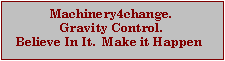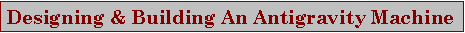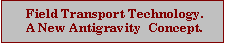What rotational rate would be required  to create the fields needed for an antigravity machine to achieve vertical acceleration.?
Only experimentation will supply the answer to this question. Based on the Earths equatorial rotational velocity, the following gives an indication of the rotational speed required. It may be that much lower rates are needed as the mass would be minimal. Also the dual rings in a counter-rotating configuration could possibly also have the effect of  lowering  the required rotational velocity.  The machine would still need to be engineered to handle at least double the estimated requirement of 460 meters per second. Roschin's machine achieved the described fields at about 30 m/sec, and the Inductrac train appears to do the same at about 1 m/sec.

Circumference of Earth at Equator =  40,076   km
Distance rotated in 1 Hour              =     1670   km
Distance rotated in 1 Minute           =       27.8 km
Distance rotated in 1 second            =  460 meters

Ring 0.265m dia  Circumference      =       0.832 m
Revs Per Second  460/0.832              =              552
Revs Per Minute  552 x 60               = 33,138 RPM

Ring 3.0 m dia  Circumference         =       9.428 m
Revs Per Second 460/9.428               =             48.8
Revs Per Minute 48.8 x 60               =    2927 RPM

As can readily be seen a small ring to test this theory cheaply, is  quite impracticable, with a RPM exceeding 33,000,  whereas a more practical size of ring at 3 meters  diameter, while rotating at a lazy 3000 RPM, would require considerable engineering skills, and cost. Dual counter-rotating rings are a requirement for stability of the machine .